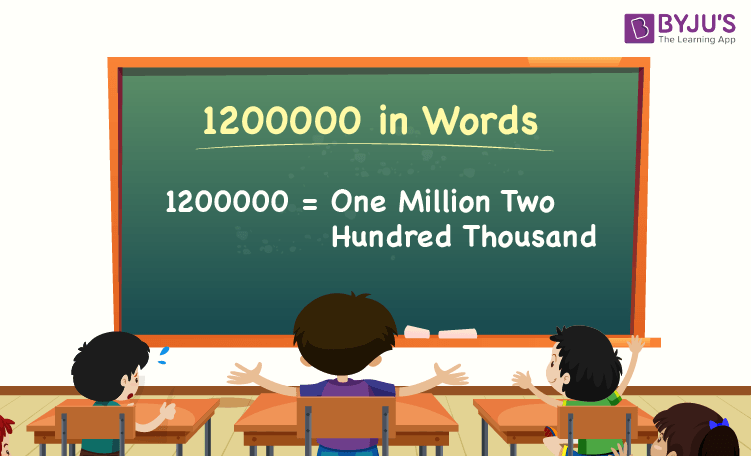# 1200000 in Words

1200000 in words is One Million Two Hundred Thousand. For example, if you earned Rs. 1200000 in a year, you can write, “I have earned Rs. One Million Two Hundred Thousand in a year”. In general, the number name of any number can be written using the ones, tens, hundreds, and thousands place of a number. Thus, the place value chart is useful for writing the number 1200000 in words.

 1200000 in Words: One Million Two Hundred Thousand

In this article, we will learn how to write 1200000 in words, and look at the solved examples in detail.

## How to Write 1200000 in Words?To write the number name of 1200000, first, we have to determine the place value of each digit of the given number 1200000. Hence, for the number 1200000,

1’s place of 1200000 is 0

10’s place of 1200000 is 0.

100’s place of 1200000 is 0.

1000’s place of 1200000 is 200.

Million’s place of 1200000 is 1.

The following chart represents the place value of the number 1200000:

 Millions Thousands Hundreds Tens Ones 1 200 0 0 0

Therefore, the number 1200000 in words is One Million Two Hundred Thousand.

### Examples

Example 1:

Find the value of 1205000 – 5000. Describe the value in words.

Solution:

Given expression: 1205000 – 5000

⇒ 1205000 – 5000 = 1200000

So, the value of 1205000 – 5000 is 1200000.

Hence, 1200000 in words is one million two hundred thousand.

Example 2:

Express the value of one million two hundred thousand minus two hundred thousand in words.

Solution:

One million two hundred thousand = 1200000

Two hundred thousand = 200000

One million two hundred thousand minus two hundred thousand = 1200000 – 200000 = 1000000.

Hence, the value of one million two hundred thousand minus two hundred thousand is one million in words.

Stay tuned to BYJU’S – The Learning App, and download the app to learn all Maths concepts quickly by exploring more learning videos.

## Frequently Asked Questions on 1200000 in Words

Q1

### Write 1200000 in words.

1200000 in words is One Million Two Hundred Thousand.

Q2

### Express the value 1000000 + 250000 – 50000 in words.

Simplifying 1000000 + 250000 – 50000, we get 1200000. Hence, 1200000 in words is one million two hundred thousand.

Q3

### How to write one million two hundred thousand in numbers?

One million two hundred thousand in numbers is 1200000.

Q4

### Is the number 1200000 an odd number?

No, the number 1200000 is not an odd number.

Q5

### Is 1200000 a composite number?

Yes, 1200000 is a composite number.

Test your Knowledge on 1200000 in Words